: 5 Radiation : Two dimensional anelastic model : 3 Turbulent parameterization

# 4 Dust transport

The spatial distribution of dust mass mixing ratio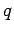is calculated by advection diffusion equation which considers gravitational settling of dust.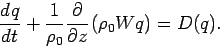(20)

The dust terminal velocity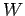is calculated as follows (Conrath, 1975).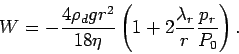(21)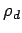is the density of dust particle,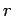is the radius of dust particle,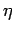is atmospheric viscosity,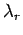is the mean free path of atmospheric gas at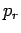. The equation (21) is applied for each dust particle with different radius. However, the size distribution of dust is not considered here for simplicity and the radius of dust particle is supposed to be equal to the mode radius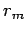of dust particle in equation (38) which is the size distribution function of dust.

It is supposed that the dust injection from the surface to atmosphere occurs when the surface stress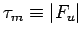exceeds some threshold value. The value of dust flux from the surface is constant.

## Parameters

 Parameters Standard Values Note3000 kgm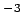Conrath (1975)1.5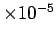kgm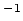sec$B!7(B25 hPa $B!7(B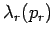2.2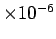m $B!7(B0.4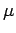m Toon et al. (1977)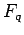3.7kgm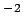White et al. (1997) (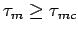)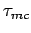0.01 Pa: 5 Radiation : Two dimensional anelastic model : 3 Turbulent parameterization Odaka Masatsugu $BJ?@.(B19$BG/(B4$B7n(B25\$BF|(B